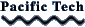Graphing Calculator Viewer allows you to view and interact with Graphing Calculator documents. It includes examples and tutorials for the software.

Version 4.0 is the latest commercial release of the original Graphing Calculator, beloved by students and teachers, which Apple bundled with the introduction of the Power Macintosh.

The story behind its development is here. It features:

• 2D curves
• 3D surfaces
• Inequalities
• Save movies
• Save for Web saves HTML & PNG files
• Save as RTF export to word processors
• Polar, cylindrical, spherical coordinates
• Define functions and variables
• Piecewise defined functions
• Complex number arithmetic
• Multiple equations
• Implicit curves and surfaces
• Parametric curves and surfaces
• Surfaces of revolution
• Contour plots
• Density plots
• Color maps
• Coordinate transformations
• Vector fields in two and three dimensions
• Curves and surfaces in four dimensions
• Direction fields
• Differential equations in two and three dimensions
• Data plots in two and three dimensions
• Functions of complex variables
• Interactive complex parameters
• Complex parametric curves
• Conformal mapping
• Complex surfaces

Take a guided tour.